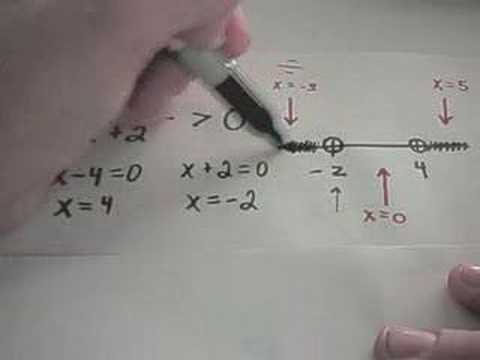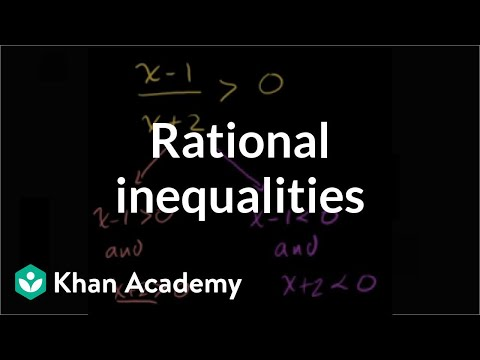# math problem help? rational abs. value inequality?

|x+7/x-3| > or equal to 2

so that's the absolute value of (x+7 divided by x-3) is greater than or equal to 2

Update:

just like to announce the first answerer is wrong, nice try though. i know you cant multiply by denom because you dont know if x is pos or neg so you dont know if the > would become a < or not.

Relevance

when you have greater, it makes an "or" value. Hint: Greataur! Just say it differently. Anyway, make two different equations, one with 2 and one with -2.

x+7 / x - 3 >= 2

Multiply by denominator.

x+7 >= 2x-6

13 >= x

x+7 / x - 3 <= -2 Notice that the sign in front of the 2, as well as the inequality has flipped.

x+7 <= -2x+6

3x <= -1

x <= -1/3

13 >=x OR x <= -1/3

•Login to reply the answers
• |(x + 7)/(x - 3)| ≥ 2

so (x + 7) / (x - 3) ≤ -2 OR (x + 7) / (x - 3) ≥ 2

{(x + 7) / (x - 3)} + 2 ≤ 0 OR {(x + 7) / (x - 3)} - 2 ≥ 2

{(x + 7) / (x - 3)} + 2(x - 3) / (x - 3) ≤ 0 OR ... do the same thing for the second possibility

[x + 7 + 2x - 6] / (x - 3) ≤ 0 OR ...

(3x + 1) / (x - 3) ≤ 0 OR ...

Now 3x + 1 = 0 when x = -1/3 OR ...

and x - 3 = 0 when x = 3 → so undefined when x = 3

Then follow the method shown here for each of the possibilities:

http://www.analyzemath.com/Rational-Inequalities/R...

Maybe this will help too:

http://tutorial.math.lamar.edu/Classes/Alg/SolveRa...

This video shows the method:and here's a video that shows two other methods you could use:•Login to reply the answers
• |(x+7)/(x-3)| ≥ 2

This means:

(x+7)/(x-3) ≥ 2, or

-((x+7)/(x-3)) ≥ 2.

case 1:

(x+7)/(x-3) ≥ 2

Multiply both sides by x-3, giving

x+7 ≥ 2(x-3), or

x+7 ≥ 2x - 6.

Subtract x from both sides and add 6 to both sides, giving

13 ≥ x, or x ≤ 13.

case 2:

-((x+7)/(x-3)) ≥ 2

Multiply both sides by -1, giving

(x+7)/(x-3) ≤ -2.

Multiply both sides by x-3, giving

x+7 ≤ -2(x-3), or

x+7 ≤ -2x + 6.

Add 2x to both sides and subtract 7 from both sides, giving

3x ≤ -1.

Solve for x:

x ≤ -1/3.

•Login to reply the answers